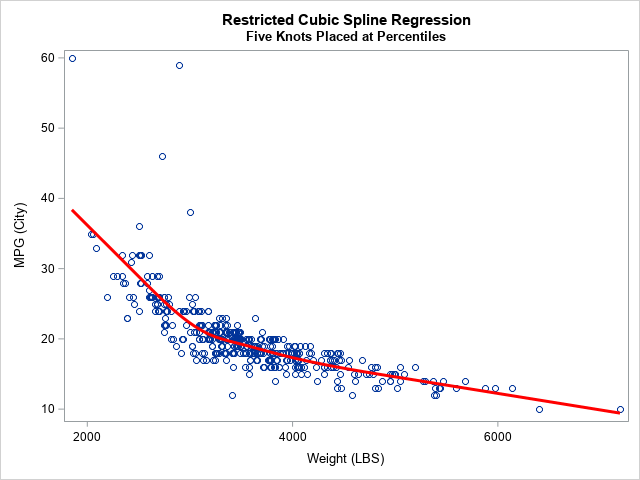Maybe if we think and wish and hope and pray
It might come true.
Oh, wouldn't it be nice?

The Beach Boys

Months ago, I wrote about how to use the EFFECT statement in SAS to perform regression with restricted cubic splines. This is the modern way to use splines in a regression analysis in SAS, and it replaces the need to use older macros such as Frank Harrell's %RCSPLINE macro. I shared my blog post with a colleague at SAS and mentioned that the process could be simplified. In order to specify the placement of the knots as suggested by Harrell (Regression Modeling Strategies, 2010 and 2015), I had to use PROC UNIVARIATE to get the percentiles of the explanatory variable. "Wouldn't it be nice," I said, "if the EFFECT statement could perform that computation automatically?"

I am happy to report that the 15.1 release of SAS/STAT (SAS 9.4M6) includes a new option that makes it easy to place internal knots at percentiles of the data. You can now use the KNOTMETHOD=PERCENTILELIST option on the EFFECT statement to place knots. For example, the following statement places five internal knots at percentiles that are recommended in Harrell's book:
EFFECT spl = spline(x / knotmethod=percentilelist(5 27.5 50 72.5 95));

### An example of using restricted cubic in regression in SAS

Restricted cubic splines are also called "natural cubic splines." This section shows how to perform a regression fit by using restricted cubic splines in SAS.

For the example, I use the same Sashelp.Cars data that I used in the previous article. For clarity, the following SAS DATA step renames the Weight and MPG_City variables to X and Y, respectively. If you want to graph the regression curve, you can sort the data by the X variable, but this step is not required to perform the regression.

```/* create (X,Y) data from the Sashelp.Cars data. Sort by X for easy graphing. */ data Have; set sashelp.cars; rename mpg_city = Y weight = X model = ID; run;   proc sort data=Have; by X; run;```

The following call to PROC GLMSELECT includes an EFFECT statement that generates a natural cubic spline basis using internal knots placed at specified percentiles of the data. The MODEL statement fits the regression model and the OUTPUT statement writes an output data set that contains the predicted values. The SGPLOT procedure displays a graph of the regression curve overlaid on the data:

```/* fit data by using restricted cubic splines using SAS/STAT 15.1 (SAS 9.4M6) */ ods select ANOVA ParameterEstimates SplineKnots; proc glmselect data=Have; effect spl = spline(X/ details naturalcubic basis=tpf(noint) knotmethod=percentilelist(5 27.5 50 72.5 95); /* new in SAS/STAT 15.1 (SAS 9.4M6) */ model Y = spl / selection=none; /* fit model by using spline effects */ output out=SplineOut predicted=Fit; /* output predicted values */ quit;   title "Restricted Cubic Spline Regression"; title2 "Five Knots Placed at Percentiles"; proc sgplot data=SplineOut noautolegend; scatter x=X y=Y; series x=X y=Fit / lineattrs=(thickness=3 color=red); run;```In summary, the new KNOTMETHOD=PERCENTILELIST option on the EFFECT statement simplifies the process of using percentiles of a variable to place internal knots for a spline basis. The example shows knots placed at the 5th, 27.5th, 50th, 72.5th, and 95th percentiles of an explanatory variable. These heuristic values are recommended in Harrell's book. For more details about the EFFECT statement and how the location of knots affects the regression fit, see my previous article "Regression with restricted cubic splines in SAS."

You can download the complete SAS program that generates this example, which requires SAS/STAT 15.1 (SAS 9.4M6). If you have an earlier release of SAS, the program also shows how to perform the same computations by calling PROC UNIVARIATE to obtain the location of the knots.

The post An easier way to perform regression with restricted cubic splines in SAS appeared first on The DO Loop.